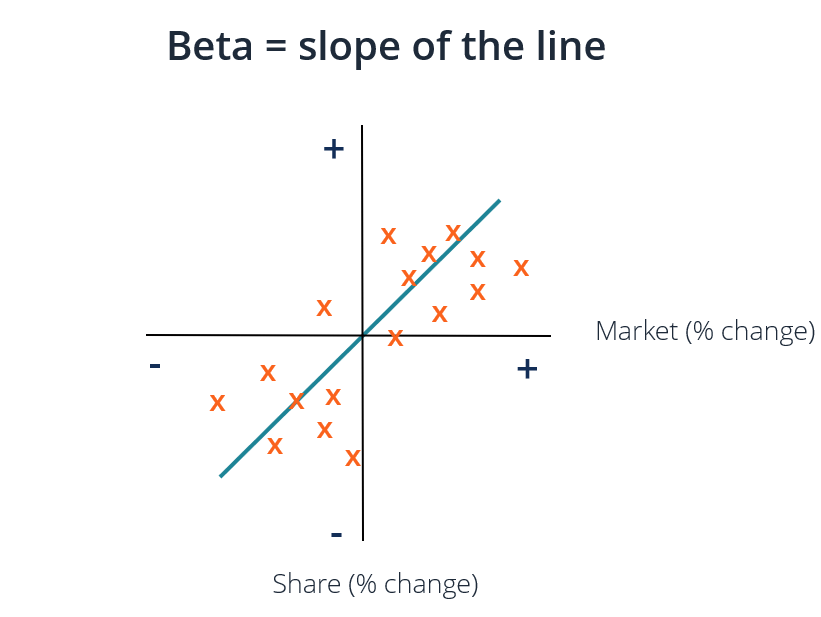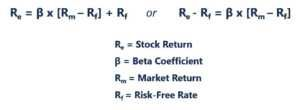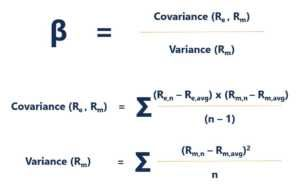# Beta Coefficient

A measure of a security's sensitivity to movements in the overall market

## What is the Beta Coefficient?

The Beta coefficient is a measure of sensitivity or correlation of a security or investment portfolio to movements in the overall market. We can derive a statistical measure of risk by comparing the returns of an individual security/portfolio to the returns of the overall market and identify the proportion of risk that can be attributed to the market.### Systematic vs Unsystematic Risk

We can think about unsystematic risk as “stock-specific” risk and systematic risk as “general-market” risk. If we hold only one stock in a portfolio, the return of that stock may vary wildly compared to the average gain or loss of the overall market as reflected by a major stock index such as the S&P 500. However, as we continue adding more to the portfolio, the portfolio’s returns will gradually start more closely resembling the overall market’s returns. As we diversify our portfolio of stocks, the “stock-specific” unsystematic risk is reduced.

Systematic risk is the underlying risk that affects the entire market. Large changes in macroeconomic variables, such as interest rates, inflation, GDP, or foreign exchange, are changes that impact the broader market and that cannot be avoided through diversification. The Beta coefficient relates “general-market” systematic risk to “stock-specific” unsystematic risk by comparing the rate of change between “general-market” and “stock-specific” returns.

### The Capital Asset Pricing Model (CAPM)

The Capital Asset Pricing Model (or CAPM) describes individual stock returns as a function of the overall market’s returns.Each of these variables can be thought of using the slope-intercept framework where Re = y, B = slope, (Rm – Rf) = x, and Rf = y-intercept. Important insights to be gained from this framework are:

1. An asset is expected to generate at least the risk-free rate of return.
2. If the Beta of an individual stock or portfolio equals 1, then the return of the asset equals the average market return.
3. The Beta coefficient represents the slope of the line of best fit for each Re – Rf (y) and Rm – Rf (x) excess return pair.

In the graph above, we plotted excess stock returns over excess market returns to find the line of best fit. However, we observe that this stock has a positive intercept value after accounting for the risk-free rate. This value represents Alpha, or, the additional return expected from the stock when the market return is zero.

### How to Calculate the Beta Coefficient

To calculate the Beta of a stock or portfolio, divide the covariance of the excess asset returns and excess market returns by the variance of the excess market returns over the risk-free rate of return:### Advantages of using Beta Coefficient

One of the most popular uses of Beta is to estimate the cost of equity (Re) in valuation models. The CAPM estimates an asset’s Beta based on a single factor, which is the systematic risk of the market. The cost of equity derived by the CAPM reflects a reality in which most investors have diversified portfolios from which unsystematic risk has been successfully diversified away.

In general, the CAPM and Beta provide an easy-to-use calculation method that standardizes a risk measure across many companies with varied capital structures and fundamentals.

### Disadvantages of Using Beta Coefficient

The largest drawback of using Beta is that it relies solely on past returns and does not account for new information that may impact returns in the future. Furthermore, as more return data is gathered over time, the measure of Beta changes, and subsequently, so does the cost of equity.

While systematic risk inherent to the market has a meaningful impact in explaining asset returns, it ignores the unsystematic risk factors that are specific to the firm. Eugene Fama and Kenneth French added a size factor and value factor to the CAPM, using firm-specific fundamentals to better describe stock returns. This risk measure is known as the Fama French 3 Factor Model.

Valuations and analysis of portfolio investments is critical work for many financial analysts. To learn more about valuation methods and analysis, the following resources may be helpful.

### Other Resources

• Valuation Methods
• Unlevered Beta
• Weighted Average Cost of Capital
• Investing: A Beginner’s Guide

### Financial Analyst Training

Get world-class financial training with CFI’s online certified financial analyst training program!

Gain the confidence you need to move up the ladder in a high powered corporate finance career path.

Learn financial modeling and valuation in Excel the easy way, with step-by-step training.